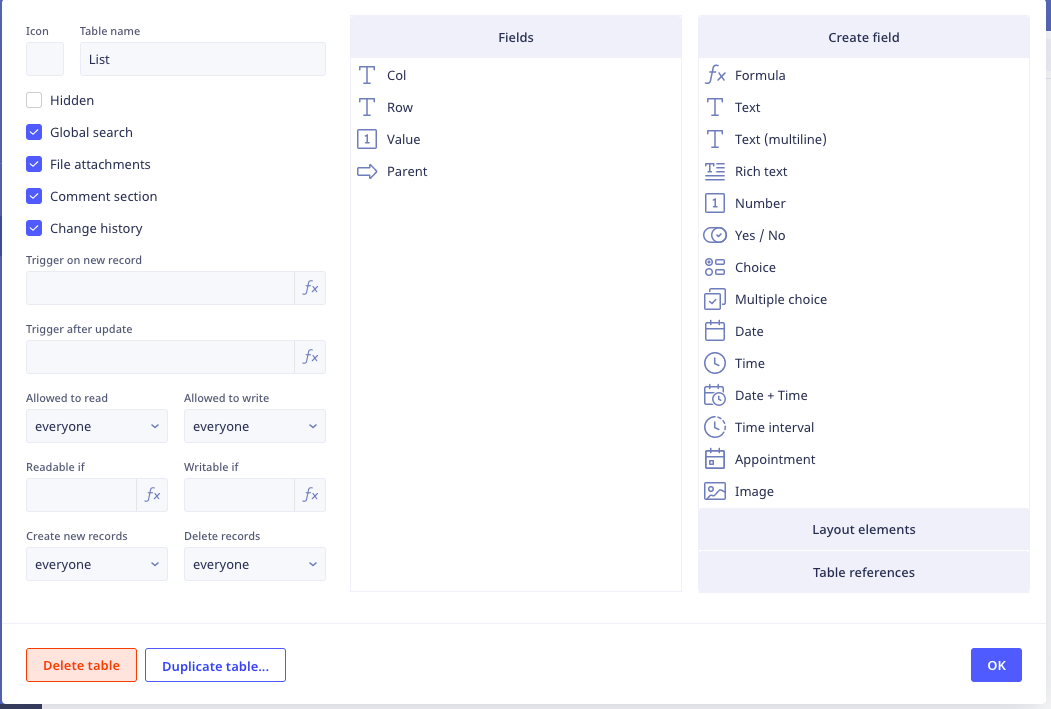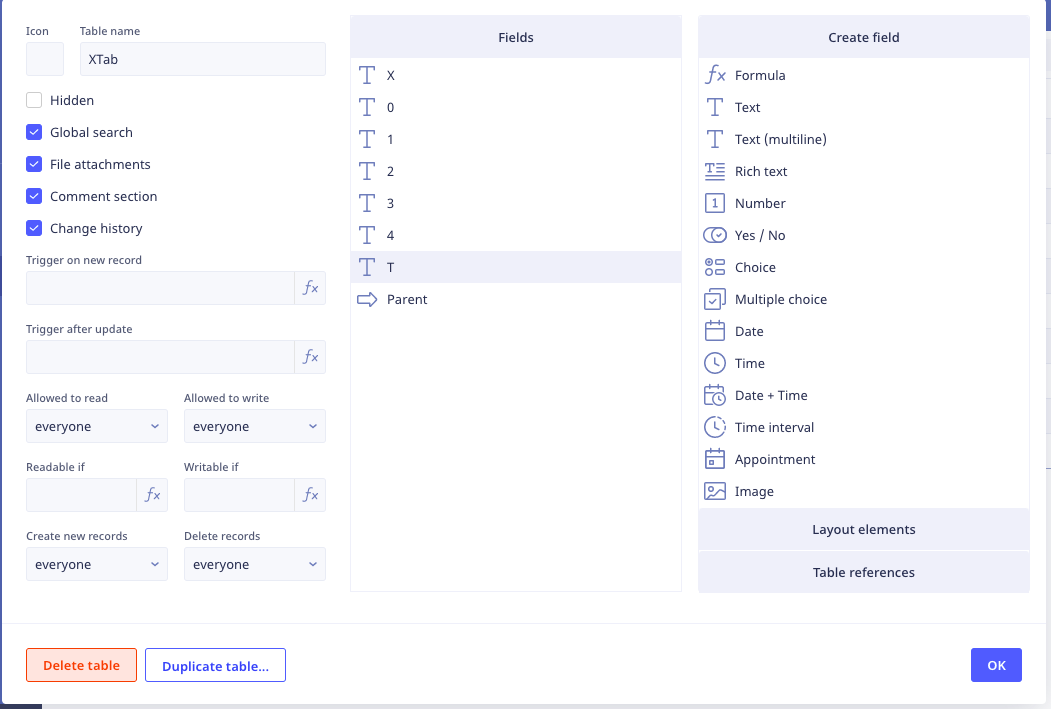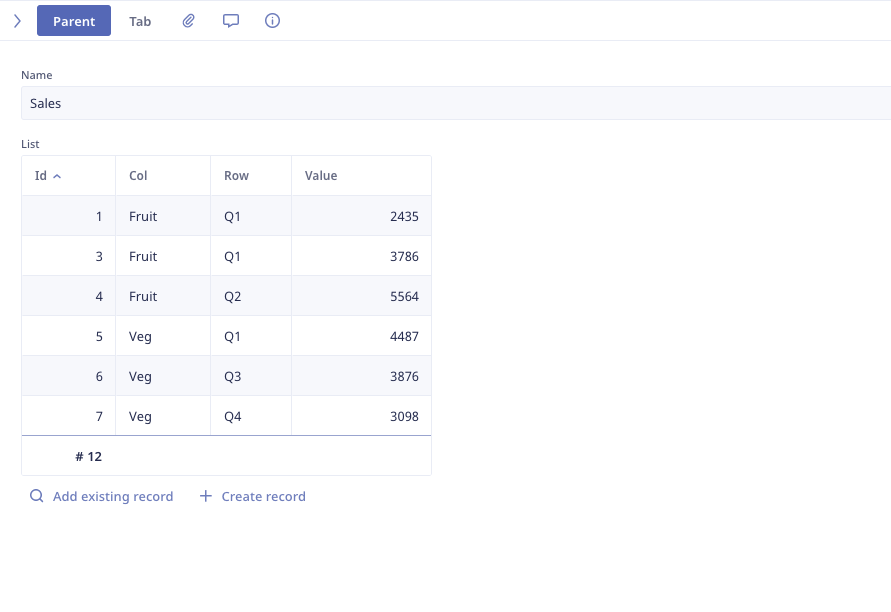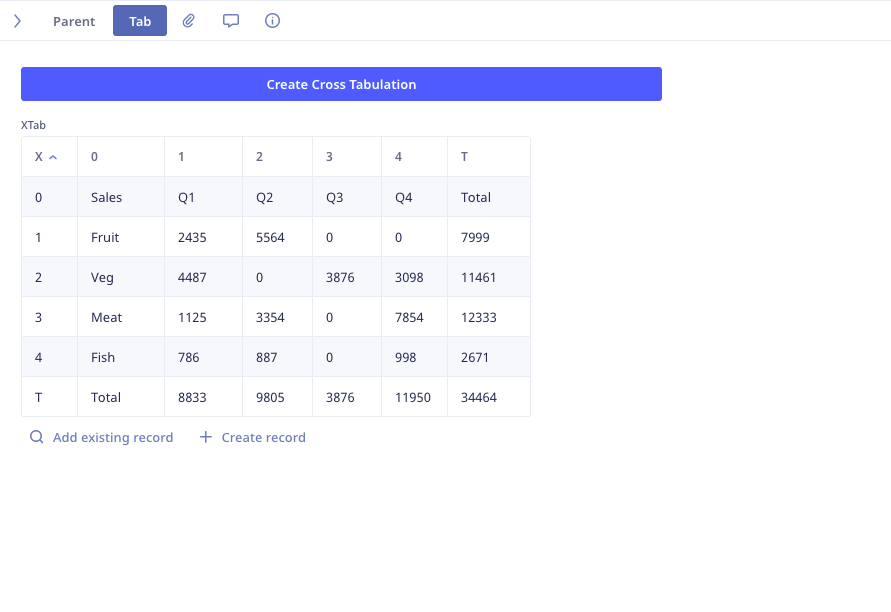1# Cross Tab in a Form

I have come up with a way to cross tabulate a table of values in a form

This is the table of valuesAnd this is the table I use to create the Cross TabThis code

``````do as server
delete XTab;
let a := this;
let b := unique((List order by Row).Row);
(create XTab).(
X := "0";
'0' := a.Name;
'1' := item(b, 0);
'2' := item(b, 1);
'3' := item(b, 2);
'4' := item(b, 4);
T := "Total";
Parent := a
);
let t1 := 0;
let t2 := 0;
let t3 := 0;
let t4 := 0;
let tt := 0;
let n := 1;
for c in unique(List.Col) do
let s1 := number(first(a.List[Col = c and Row = item(b, 0)]).Value);
let s2 := number(first(a.List[Col = c and Row = item(b, 1)]).Value);
let s3 := number(first(a.List[Col = c and Row = item(b, 2)]).Value);
let s4 := number(first(a.List[Col = c and Row = item(b, 3)]).Value);
let st := s1 + s2 + s3 + s4;
(create XTab).(
X := n;
'0' := c;
'1' := s1;
'2' := s2;
'3' := s3;
'4' := s4;
T := st;
Parent := a
);
t1 := t1 + s1;
t2 := t2 + s2;
t3 := t3 + s3;
t4 := t4 + s4;
tt := tt + st;
n := n + 1
end;
(create XTab).(
X := "T";
'0' := "Total";
'1' := t1;
'2' := t2;
'3' := t3;
'4' := t4;
T := tt;
Parent := a
)
end
``````

Turns thisInto thisRegards John

19replies Oldest first
• Oldest first